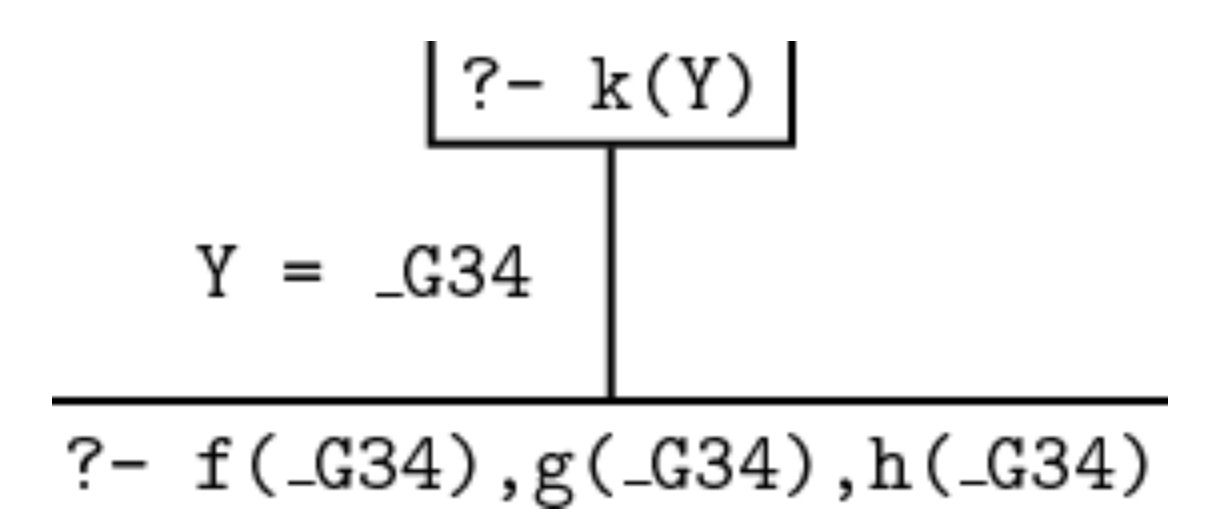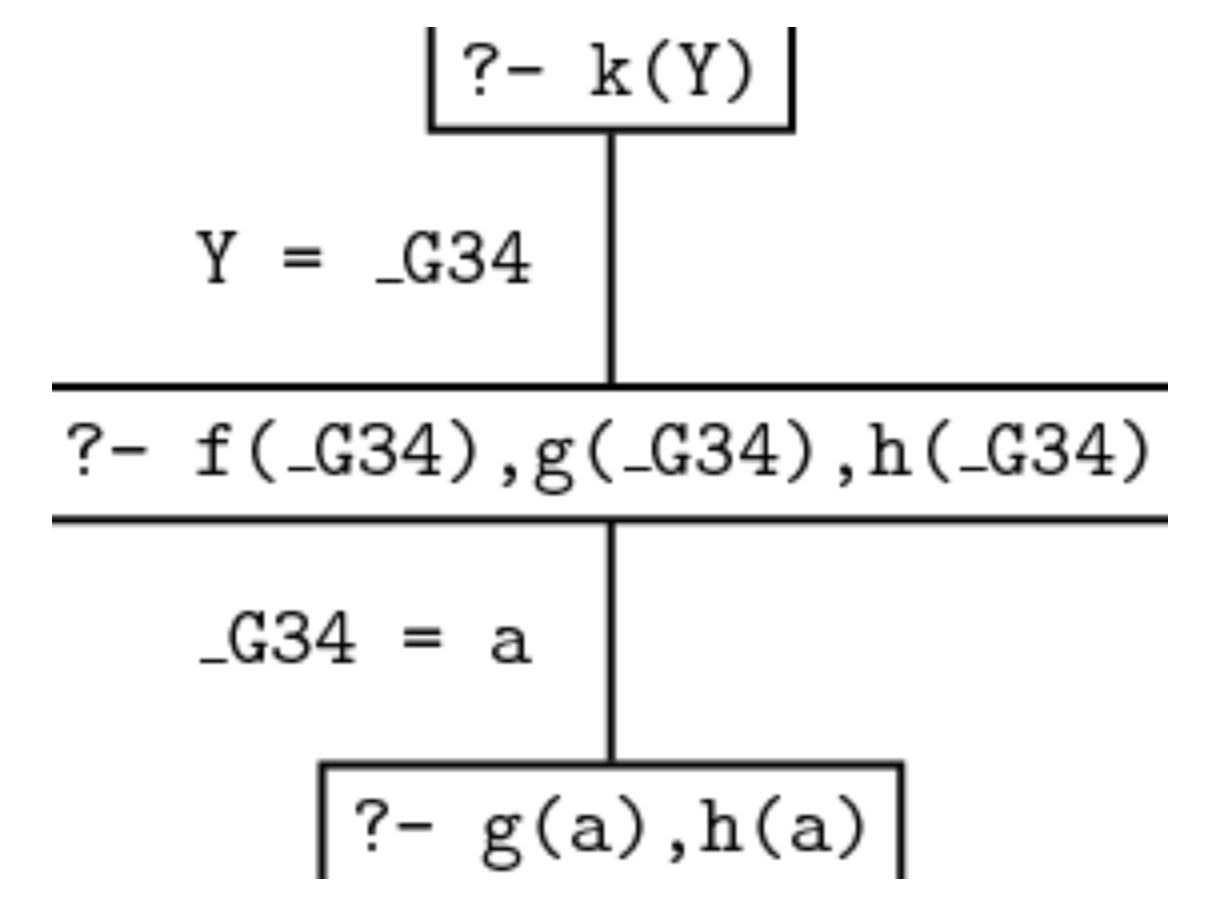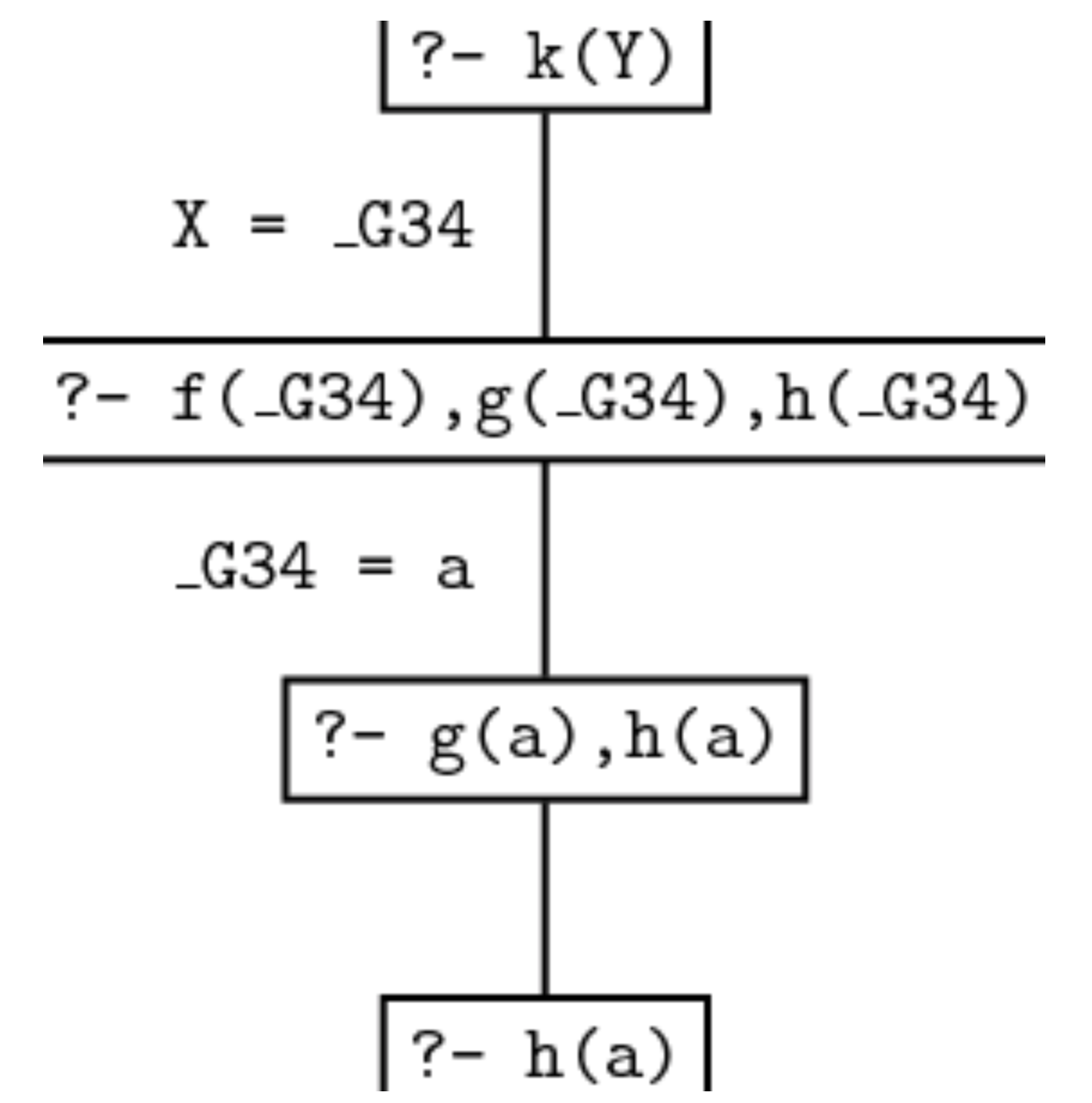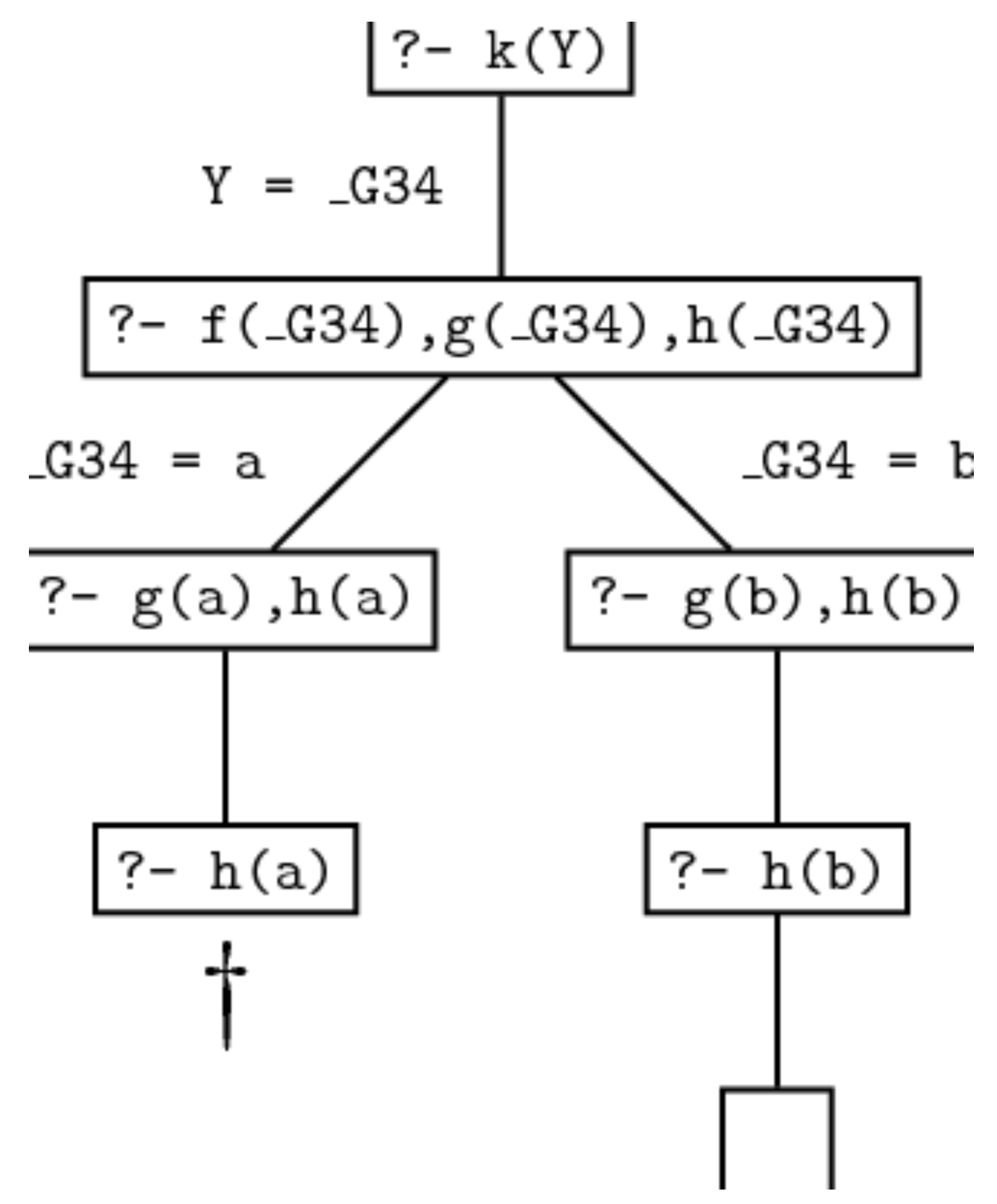# Prolog 学习 Ch2

## Unification & Proof Search

Posted by R1NG on October 12, 2021 Viewed Times

# Prolog 入门: 联合与证明搜索

## 1. 联合

1. 它们或是相同的项
2. 或这些项中包含了可以 (同时) 通过与项一并实例化使得这两个项相同的变量.

Prolog 中, 我们称两个项可被联合, 当且仅当它们同时满足下述三条规则:

1. 若两个项均为 常量 (Constants), 当且仅当它们为同一个原子或同一个数字时, 两项联合.
2. 项1 为变量而 项2 为任何类型的项, 则两项联合,且此时变量被实例化为 项2, 反之亦然. 若两项均为变量, 则在联合过程中被互相实例化. 称它们的值共享. (share values).
3. 若两项均为复杂项, 则其联合当且仅当:
3.1 函子和元 (functor and arity) 相同.
3.2 所有对应的参数都可联合.
3.3 变量实例化过程是无冲突的, 例如不存在 “必须让某个变量同时在两个不同的位置上取不同的值才能满足对应参数可联合” 的情况.

1
2
3
4
5
% a rather unnatual notation
?- =(axton, axton).

% the most common one: infix notation
?- axton = axton.


1
2
3
4
5
6
7
8
9
10
11
% fits clause 1
?- axton = axton
true.

% fits clause 1: both terms are numbers
?- 114514 = 114514.
true.

% doesn't fix: atom 'axton' and 'ryan' are different
?- axton = ryan.
false.


1
2
3
4
% quirk: prolog will consider any atoms of the form
% 'symbols' as the atom of form symbols.
?- 'axton' = axton.
true.

1
2
3
4
5
6
7
8
9
10
11
12
13
14
15
% clearly the variable can unify with the constant following clause 2.
?- axton = X.
true.

% now consider complex terms.
?- k(s(g), Y) = k(X, t(k)).
true.
% clearly it's true as long as s(g) = X, and Y = t(k),
% which follows clause 3.

% the last example
?- loves(axton, phigros) = loves(X, X).
false.
% this will not work, as it violates clause 3: the cariable instantiation is incompatible.


1
2
3
?- X = axton(X).
X  =  axton(axton(axton(axton(...))))))))
yes.


## 2. 证明搜索

1
2
3
4
5
6
7
8
9
10
11
12
13
% facts for f()
f(a).
f(b).

% facts for g()
g(a).
g(b).

% facts for h()
h(b).

% predifine a rule
k(X) :- f(X), g(X), h(X).


Prolog 对问题的求解流程基本上是基于给定的知识库, 自顶而下地尝试将用户的查询语句和知识库中的某条事实或定义的某个规则相联合. 显然, 在当前考虑的例子中, Prolog 只能将查询语句 k(Y) 和 规则 k(X) :- f(X), g(X), h(X). 联合.

1
k(_G34)


1
k(_G34)  :-  f(_G34),  g(_G34),  h(_G34).1
f(_G34),g(_G34),h(_G34).